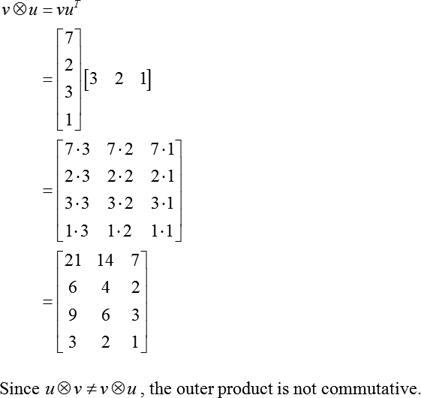# (SOLVED) Outer Product

Discipline: Mathematics

Paper Format: APA

Pages: 1 Words: 275

Question

Outer Product

The tensor product of two coordinate vectors is termed as “Outer product”. This is a special case for “Kronecker product of matrices”. Let u and v be vectors. Then, the outer product of u and v is w=uvT. The outer product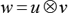is same as the matrix multiplication uvT also u is denoted by m × 1 column vector and v is denoted by n × 1 column vector.

Let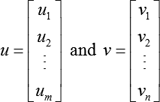be two vectors. Then, the outer product of u and v is obtained as follows: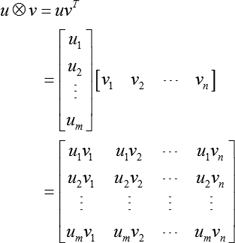Properties of an outer product:

• The result of an outer product is m × n rectangular matrix.

• The outer product is not commutative. That is,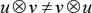• Multiply the second vector v with the resultant product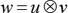gives a vector of the first factor u scaled by the square norm of the second factor v. That is,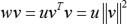Example:

Consider the vectors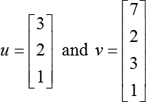.

Transpose of v is, vT = [7 2 3 1].

The outer product of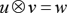, which is obtained below: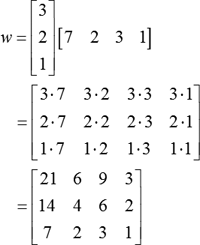Thus, the outer product is a rectangular 3 × 4 matrix.

Check the outer product is commutative or not.